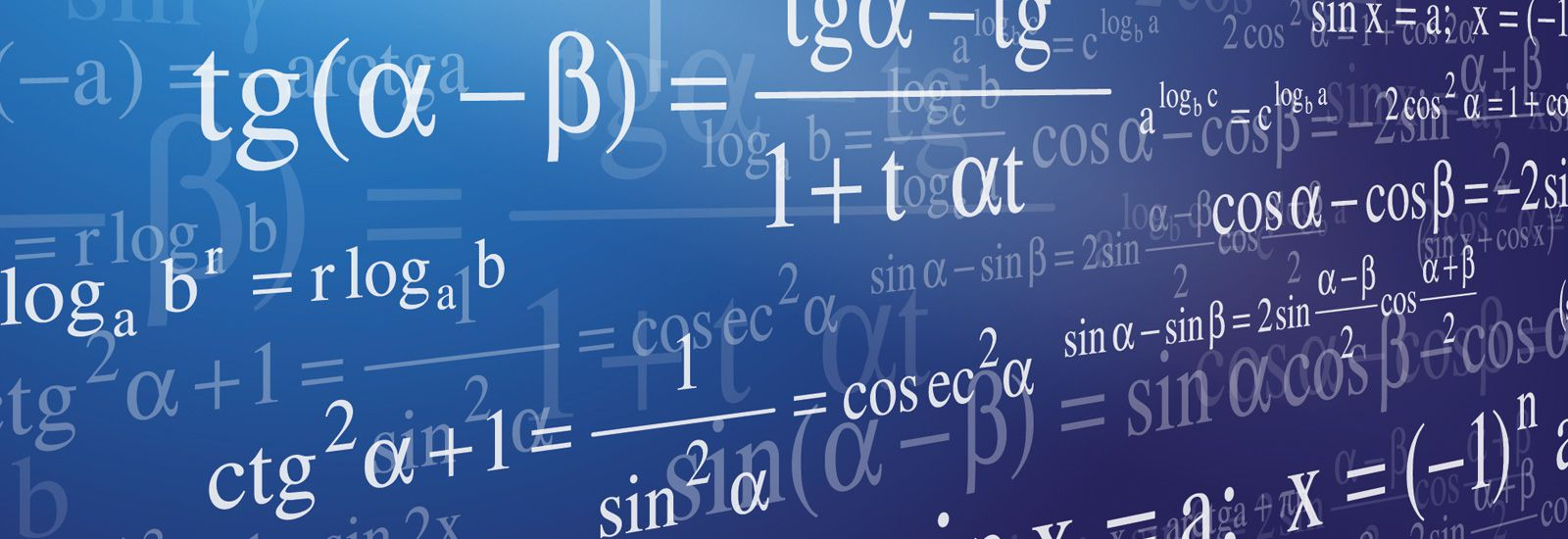# M​athematics

#### Mrs Ibbs (Head of Mathematics)

 The Team Mr Freestone (Deputy Head of Mathematics) Mr Elkington Mrs Lund Miss Arrowsmith Mrs Fletcher Mr Phillips Mrs Gill Mr Lloyd (Deputy Head of Mathematics) Mrs Rodi (Maths Intervention Tutor) Mrs Barker (Maths Intervention Tutor)

#### Introduction:

Mathematics at Thomas Alleyne’s is taught in 8  dedicated maths classrooms,  all of which have interactive whiteboards.

## Year 9

Students in Year 9 study Number, Algebra, Ratio & Proportion, Geometry & Measures, Statistics and Probability. These areas of study are spread over 20 units and students are assessed every half term. In addition to the half termly assessments there is an end of year exam in the summer term. All students receive 7 hours of maths lessons per fortnight.

## Year 10

Students in Year 10 are following the new AQA syllabus (8300). They will extend their understanding of Number, Algebra, Ratio & Proportion, Geometry & Measures, Statistics and Probability. They are assessed every half term and will receive an end of year exam in the summer term. All students receive 8 hours of maths lessons per fortnight.

## Year 11

Students in Year 11 continue to follow the new AQA syllabus (8300).  They will extend their understanding of Number, Algebra, Ratio & Proportion, Geometry & Measures, Statistics and Probability. They will complete regular assessments including a mock exam before  Christmas. All students receive 8 hours of maths lessons per fortnight.

## Year 12

A-Level Mathematics - students follow the new Edexcel 2 year linear A-Level course.  Students will study pure mathematics, mechanics and statistics. They are assessed every half term and will receive an end of year exam in the summer term. Students will receive 9 hours of maths lessons per fortnight.

A-Level Further Mathematics – students follow the new Edexcel 2 year A-Level course. Students will study further pure, mechanics and decision mathematics. They are assessed every half term and will receive an end of year exam in the summer term. Students will receive 7 hours of maths lessons per fortnight.

## Year 13

A-Level Mathematics - students follow the new Edexcel 2 year linear A-Level course.  Students will study pure mathematics, mechanics and statistics. They are assessed every half term with mock exams before Christmas.  Students will receive 9 hours of maths lessons per fortnight.

A-Level Further Mathematics – students follow the new Edexcel 2 year A-Level course. Students will study further pure, mechanics and decision mathematics. They are assessed every half term with mock exams before Christmas.  Students will receive 7 hours of maths lessons per fortnight

Extra-Curricular:   The department arranges various extra-curricular activities including entry to the UK Maths Challenge competitions,  the House Chess competition and trips to the Maths Inspiration lectures.

### Equipment:

Students are expected to bring the following equipment to all Mathematics lessons:

Black Pen

Pencil

Ruler

Protractor

Compass

Calculator

(We recommend the Casio fx-83 GTX)

Green Pen

All equipment is available to purchase from the Mathematics department  and the Thomas Alleyne’s online shop.